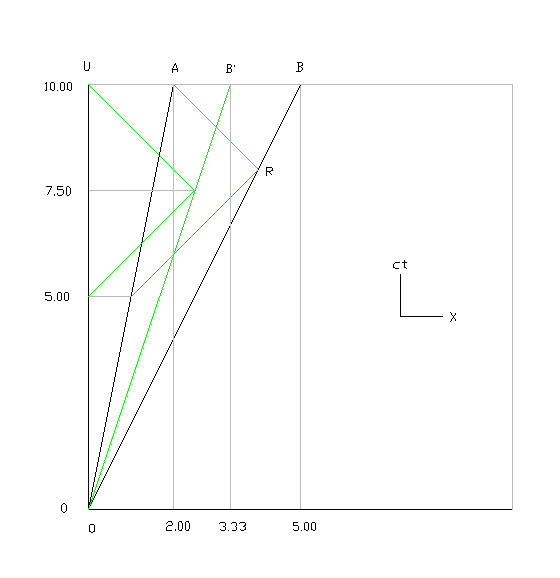# Relative velocities In Space-Time Diagram

Quarlep
How can we show one dimensional relative paralel velocities in space time diagram ?
(One dimensional means theres only pozitive and negaive values of speed)

Gold Member
On a space-time diagram (t,x) the velocity relative to this coordinate system (t,x) of a world line is simply the slope of the world line, where 45 degrees is the speed of light (units c=1). If one has 2 world lines, one can measure each line's slope to get each world line's velocity relative to the coordinate system (t,x). If one wants to get then the relative velocity of one world line to the other, one can use the relativistic velocity addition formula on the 2 previously obtained velocities. I'm not sure if there is a direct way of obtaining the relative velocity simply by looking at the two world lines in a space-time diagram.

Gold Member
How can we show one dimensional relative paralel velocities in space time diagram ?
(One dimensional means theres only pozitive and negaive values of speed)
Here's a space time diagram showing 10% increments of speeds starting with -90%c and going to +90%c. There are also two light rays at -c and +c shown in red: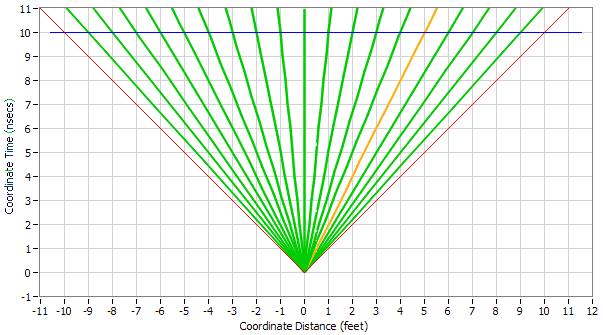I also drew in a horizontal blue line at the Coordinate Time of 10 nanoseconds so that you can easily determine the speed of any of the green lines by looking down at the corresponding Coordinate Distance. Note that light travels at 1 foot per nanosecond.

Is that the sort of answer you were looking for?

Quarlep
Let me be clear Lets suppose we are observing to objects one of them is moving 0.5c and the other one 0.2 c so there is relative velocity (Supoose these are the vectors and they are parallel)

Quarlep
I know it but I want to show it in space time diagram

Gold Member
I know it but I want to show it in space time diagram

What do you mean by "show it" in space time diagram? ghwellsjr has already shown them to you. Each of the lines in his space time diagram represents a different object moving at a different speed, up to light traveling at "c" for the red lines.

Gold Member
Let me be clear Lets suppose we are observing to objects one of them is moving 0.5c and the other one 0.2 c so there is relative velocity (Supoose these are the vectors and they are parallel)
If we just pick out the two lines for those speeds in my diagram from post #3, there won't be any obvious way to see or determine the speed of one of them relative to the other but if we add in markers (dots) for the Proper Time of one of them, say the one that is traveling at 0.2c, then we can show how it can establish the speed of the other one by sending light signals to the other one and timing the reflection. This works just like a laser range finder except that it also registers the time when the signal was sent and when the reflection was received. Here is a spacetime diagram showing the process: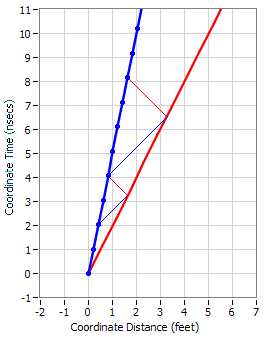The blue object sends out its first signal (in blue) at its time of 2 nanoseconds and it receives the reflection (in red) at its time of 4 nanoseconds. It assumes that the signal took the same amount of time to get to the red object as it did for the reflection to get back and that it traveled at c, one foot per nanosecond, so it places the red object at 1 foot away at its time of 3 nanoseconds. Do you understand the math or do you want me to go over it?

Then the blue object sends out its second signal at its time of 4 nanoseconds and it receives the reflection at its time of 8 nanoseconds. So it establishes that the red object was 2 feet away at its time of 6 nanoseconds. Now it can establish the speed of the red object relative to itself by taking the differences between the distances divided by the differences between the times. Its calculation is:

(2-1)/6-3) = 1/3 = 0.3333c

If you check the relativistic velocity addition formula, you will see that this is correct. That calculation is:

(0.5-0.2)/(1+(0.5)(-0.2)) = 0.3/(1-0.1) = 0.3/0.9 = 0.3333

We can also transform from the above IRF to the one in which the blue object is at rest and we directly see that the red object is traveling at 0.3333c relative to the blue object: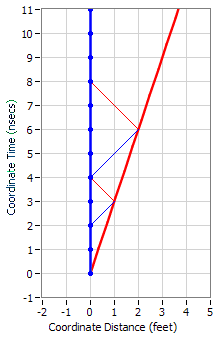Note that it doesn't matter when the blue object sends out its signals, the final answer will always be the same. And we could have done the exercise from the point of view of the red object; it, too, would establish that the blue object was moving away from it at 0.3333c.

•Matterwave
Gold Member
And we could have done the exercise from the point of view of the red object; it, too, would establish that the blue object was moving away from it at 0.3333c.
In fact, here are the diagrams showing how the red object would establish the speed of the blue object.

First, in the original IRF in which red is traveling at 0.5c and blue is traveling at 0.2c: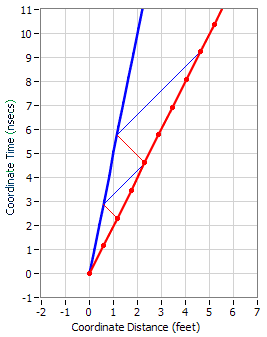And second, the IRF in which the red object is at rest: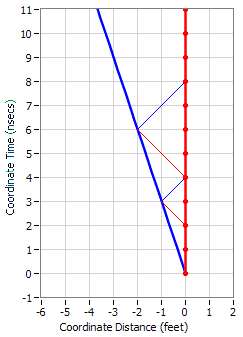Gold Member
In fact, here are the diagrams showing how the red object would establish the speed of the blue object.

First, in the original IRF in which red is traveling at 0.5c and blue is traveling at 0.2c:

And second, the IRF in which the red object is at rest:

I'm curious ghwells, do you have a program to generate these diagrams, or do you make them "by hand" for each thread? That would seem really tedious. haha

Staff Emeritus
Let me be clear Lets suppose we are observing to objects one of them is moving 0.5c and the other one 0.2 c so there is relative velocity (Supoose these are the vectors and they are parallel)

I'm not sure I follow the question.

If you are observing two objects, one of which is moving at .5c and another at .2c, the velocities of the two objects are not parallel. You write "suppose these are the vectors and they are parallel". Interpreting "these" as the velocities of the two objects, they are not parallel, you cannot "suppose" that they are.

Parallel lines must have the same slope - parallel velocities must have the same velocity.

If you draw a third object moving at .5c, but at a different position than the first object, the first and third objects velocities will be parallel
If you draw a fourth object moving at .2c, but at a different position than the second object, the second and fourth object will have parallel velocities.

You can use the concept of rapidity http://en.wikipedia.org/wiki/Rapidity to get a measure of velocity that you can add or subtract, this is additive in the same way that angles usually add it Euclidean geometry.

Using the concept of rapidity, you can say that the rapidity between the first object and the second object is equal to the sum of the rapidities of the two objects relative to yourself.

wiki said:
the rapidity φ corresponding to velocity v is φ = arctanh(v / c).

here arctanh is the inverse hyperbolic tangent, see for instance http://mathworld.wolfram.com/InverseHyperbolicTangent.html. arctanh and ##\tanh^{-1}## are different notation for the same function.

The wiki article contains a proof that rapidities add, which would be one way of describing how the velocity composition rule in special relativity is derived. The proof is based on the Lorentz transform between different frames. The proof may or may not seem satisfying to you, I don't know. To do any sort of proof, I believe you will need some familiarity with the Lorentz transform - just being able to draw a space-time diagram is probably not sufficient, I believe that you need to understand the mathematics of how one transforms between frames before you can derive the velocity composition rule.

Gold Member
I'm not sure I follow the question.

If you are observing two objects, one of which is moving at .5c and another at .2c, the velocities of the two objects are not parallel. You write "suppose these are the vectors and they are parallel". Interpreting "these" as the velocities of the two objects, they are not parallel, you cannot "suppose" that they are.

Parallel lines must have the same slope - parallel velocities must have the same velocity.

I believe the OP means that the velocities vectors themselves are parallel in real space (i.e. the two objects are moving co-linearly), not that the lines in the space-time diagram are parallel.

Homework Helper
Gold Member
By "parallel", the OP probably means something along the lines of
[the usual situation in which] "the spatial-components of their velocities are parallel (say) in their x-directions".

In the spacetime viewpoint,...
their 4-velocities [or their worldlines] are (as you say) not parallel [in spacetime].
(In addition, their spatial-x axes are not parallel [in spacetime].)

To capture the probable intention of the OP as "spatial-components are parallel", it's probably best to think:
the "tx-diagram of observer 1" is coplanar with the "t'x'-diagram of observer 2"... so you can draw the observers on the same (1+1)-dim spacetime-diagram.
[You can think this way... the y-axes of these two observers coincide (as well as for the z-axes).. but, because of their relative-motion along their x-axes, their t-axes don't coincide and their x-axes don't coincide. However, both of their tx-planes are Minkowski-orthogonal to their coinciding y- and z-axes.]

Technically speaking,
according to obs-1,
the spatial-component of the obs-2's 4-velocity
is parallel to the spatial-x-axis of obs-1... and similarly for obs-2.

Last edited:
Homework Helper
Gold Member
From the spacetime diagram above,
with the two radar times since their meeting event (at t=0): we have t=4 (send) and t=8 (receive),
the relative speed is (dx/dt)= ( (8-4)/2 )/( (8+4)/2 )=4/12=1/3
and their doppler factor is sqrt(8/4).... all in the spirit of Radar Measurements and the Bondi k-calculus.

Check that sqrt(8/4)=sqrt( (1+(1/3))/(1-(1/3)) ) [copy-paste the RHS into a google search].

Gold Member
I'm curious ghwells, do you have a program to generate these diagrams, or do you make them "by hand" for each thread? That would seem really tedious. haha
I wrote my own program to draw the diagrams but sometimes I have to edit them "by hand" in Paint.

Gold Member
I wrote my own program to draw the diagrams.
Haha nice! :D

Staff Emeritus
I believe the OP means that the velocities vectors themselves are parallel in real space (i.e. the two objects are moving co-linearly), not that the lines in the space-time diagram are parallel.

Hmmmm.

How can we show one dimensional relative paralel velocities in space time diagram ?
(One dimensional means theres only pozitive and negaive values of speed)

Since he also specified there was only one spatial dimension, the velocities should be auotmatically parallel in the sense of being colinear (as there is only one line for them the objects move on, that being the spatial axis). That doesn't necessarily rule out that that's what he might nave meant, though.

Quarlep
By parallel veloities I mean one dimensional space and theres velocity vectors so they must be parallel each other
Post 8 explains everyting to me. But I didnt understant the math of explaining these relativ velocity.

(2-1)/6-3) = 1/3 = 0.3333c

phyti
By parallel veloities I mean one dimensional space and theres velocity vectors so they must be parallel each other
Post 8 explains everyting to me. But I didnt understant the math of explaining these relativ velocity.
In the graphic, A and B are moving in the same direction, with just enough room to pass each other. Their speed relative to U is a=.2c and b=.5c respectively, with c = 1. Times are from the U clock. Speed calculation requires a distance interval and a time interval. This example is simplified by using a coincident location at t=0. A gets the 2nd position by emitting a signal (blue) at t=5, which reflects at R, and is detected at t=10.00. Per SR, A calculates the distance to R as equal path lengths (green) giving R'=(10.00-5.00)/2 = 2.50. Then A calculates the speed of B as 2.5/7.5 =.33.

Since both x and t are scaled by 1/gamma, the speed can be calculated using A or U time.

In using this procedure, it is simplest to draw the blue signal in reverse order, starting at t=10.

Using v=(b-a)/(1-ab), gives v=.3/.9=.33.